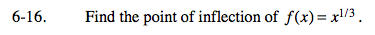### Home > CALC > Chapter 6 > Lesson 6.1.1 > Problem6-16

6-16.f ''(x) = 0 and f ''(x) = DNE will identify CANDIDATES for points of inflection.
To determine if a candidate is the location of the POI, you could either:
1. Evaluate f ''(x) before and after x = candidate. If there is a sign change, then the candidate is the x-value of a POI.
2. Evaluate f '''(candidate). If the 3rd-derivative does not equal zero, then the candidate is the x-value of a POI.

POI at (0,0).
Be sure to show all steps and justification/tests.

Use the eTool below to visualize the problem.
Click the link at right for the full version of the eTool: Calc 6-16 HW eTool